# Dirk Bertels

• Music, Maths, Science and GIC (General Idle Contemplation)
The greatest malfunction of spirit
is to believe things (Louis Pasteur)

# Constructing the Pentagon

### Introduction

The act of constructing pentagons is quite revealing, it makes one think back to the ancient Greek civilisation where philosophers like Plato and Pythagoras used geometry to explain and visualise fundamental concepts.

I will describe 3 systems of construction, all using only a set of compasses and a ruler. No metric measurements need to be made. Before describing the first method however, we need to be able to construct a right angle and a square.

The relationship between PHI and the Pentagon is touched upon in the article PHI elsewhere in this category.

### Creating a right angle

• Draw a line and choose a point C outside this line.
• With C at its centre, draw a circle that cuts the line at two points A and B.
• From one of those intersecting points (say B), draw a line through C intersecting the circle at D. Connect A to D.### Creating a square

• Using our right-angle from above, choose a point P on one of the lines. This will determine the length of one side of the square
• Using A as center, draw a circle through P creating point Q.
• Using the same radius draw little lines with compasses from P and Q, so they cross thus forming the last point R.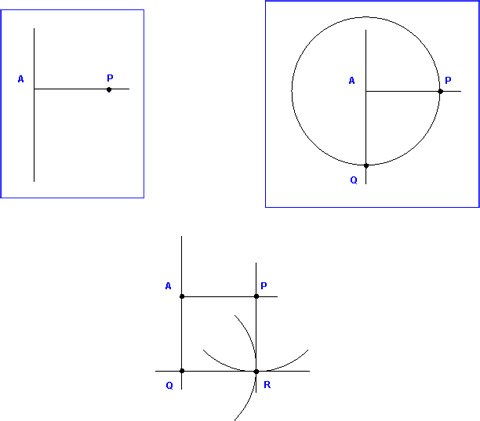### Creating a Pentagon - Version one

• Construct the square and create another one on top of it by extending AQ and PR, and using the compasses set to one of the square's sides, mark two points, one from A, the other one from P.
• Draw a circle like in the figure, still using the same radius (side of the square) and starting from center C.
• Next draw a line joining two extreme corners of the double square. Draw points A and V.
• With compasses width set to AV, draw D from A.
• From V, draw an arc with radius VD thus creating W and X.
• Keeping the same radius draw 2 more points, one from W (Y) and one from X (Z).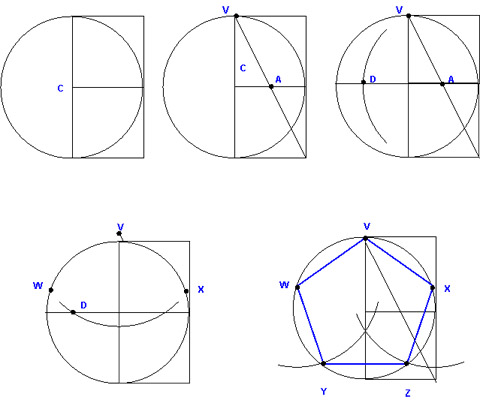### Creating a Pentagon - Version two

Note: Replace C in the first 3 figures with O.

• Draw a circle with a line through its center, and mark points A, O, and B.
• Construct a square on AB using the method described above: Choose a point outside the line, construct a right angle and after that construct the square, using AB as a measure.
• Mark points H and K using radius OC or OD from center O.
• With radius HB, find E, using both A and B as centers.
• Again with A and B as centers, find F and G using radius AB.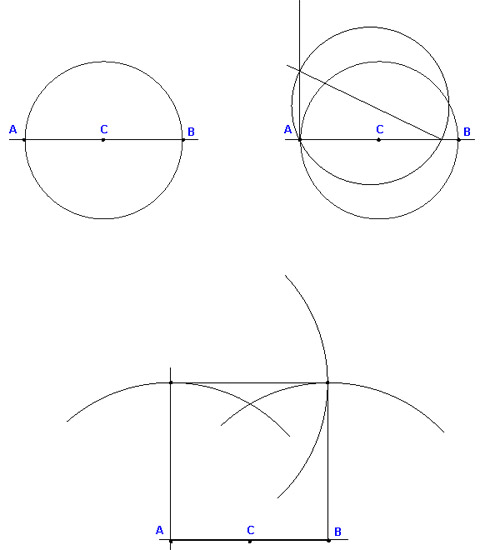### Creating a Pentagon - Version three

This is possibly the simplest one. It is my favourite method as it is easy to remember and the resulting drawing has a somewhat 'organic' look about it.
As a bonus, it also draws the triangle and square in the process. Only one setting is needed for the compasses. This setting (radius) is also the size of all edges of those figures.

• Choose a radius and draw 3 circles as shown.
• Draw the vertical line in the middle, using the intersection of 2 circles.
• Draw the axes using the found intersections.
• Draw an arc from P, use it to 'cap' the figure. This is an optional step since the points thus created are only needed to draw the square.
• Still using the same radius, draw 2 arcs from A and B to find C. Connect the dots ...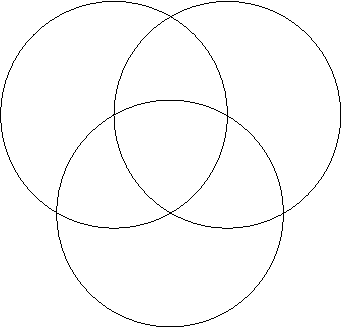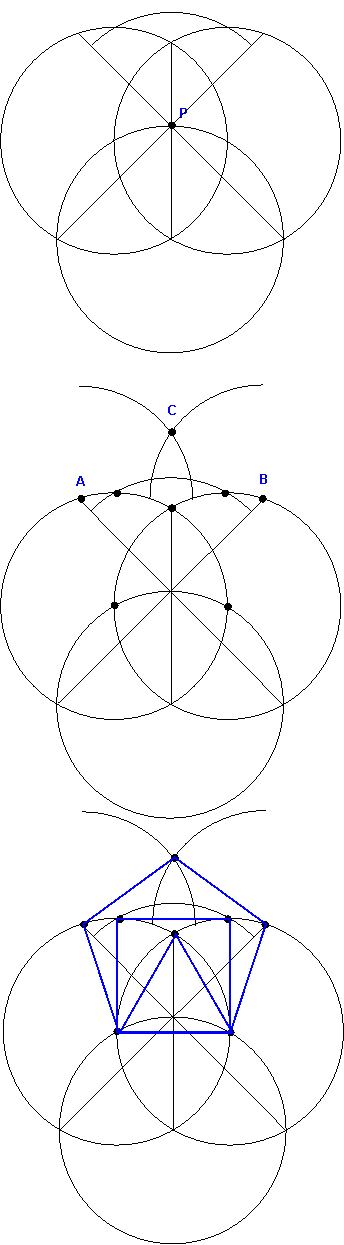### Notes

These constructions are only examples; there are a vast variety of constructions out there, depending on the approach taken. In the last example, the size of the pentagon had already been decided. It shows that by means of superimposing circles with the same diameter and connecting the newfound intersections, we can derive various polygons. It can be shown that many polygons can be constructed this way (see "The sacred art of geometry" pp34 - sorry, lost the book's details - let me know if you have them).

Following figure uses the points derived from the diagram above and 'connects the dots' - the result has a well-balanced look about it.### The Pentagon Knot

Cut a paper strip and knot it once, in the standard manner. The result will be a pentagon. This seems significant - though I can't quite put my finger on the reason why. I just know that the mathematical study of knots is a branch of topology.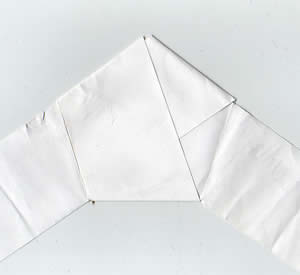figure: the Pentagon Knot

Interestingly, though we are digressing here, the study of knots is also used in combination with another seemingly simple entity, the soap bubble. Soap bubbles are studied by mathematicians because the bubbles sticking together take such a form that the total surface of the spherical segments, and the common part to both is the smallest possible surface which will contain the quantities of air and keep them separate. Now, mathematicians sometimes make knots out of wire and dip them into a bubble-making solution. They then carefully examine the way the soap film clings to the wire. The form of the stretched and twisted soap film is called a Seifert Surface. There's much on Knot Theory on the web, try Wikipedia's page for starters.